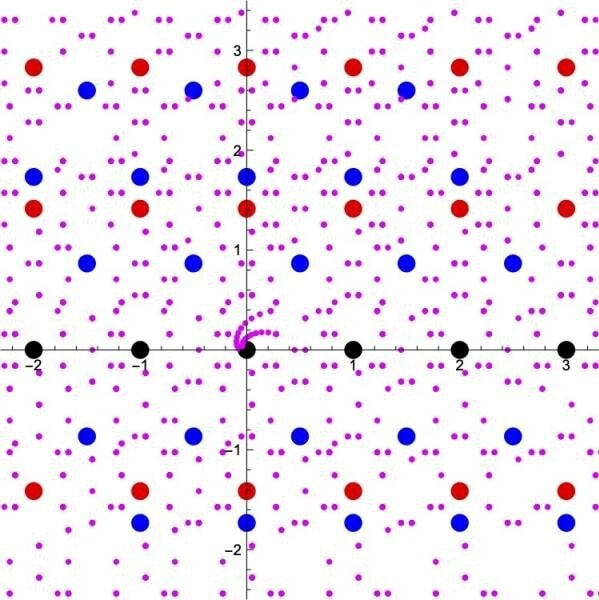# Researchers discovered link between complex numbers and superstring theory

Number theory is a branch of mathematics studying the properties of whole numbers. It is an active area of ​​research in fundamental mathematics because it is located at the interconnection of all other disciplines. By precisely analyzing a particular analytical function of the theory, the modular form, a duo of researchers showed that in certain cases, this function could be described by means of quantum observables in the theory of superstrings. A result that could help confirm certain properties of this theory.

A collaboration of a mathematician and a physicist has shown that the modular forms associated with elliptic curves with complex multiplications are expressed in terms of observables in superstring theory. The study was published in the journal Communications in Mathematical Physics.

The concept of numbers can be extended from integers and rational numbers to include all real numbers and complex numbers, all at once.

But it is also possible to extend the concept gradually, by adding the roots of polynomials with rational number coefficients (such as the square root of 2 and the square root of 3) little by little. This special class of complex numbers are referred to as "numbers." The precise details of how the concept of numbers can be extended has been considered as one of the important themes in number theory.Extension of the concept of integral “numbers”. Black dots are the ordinary integers represented in a complex plane. Adding or multiplying any pair of black dots results in another black point. All the red dots and the blue dots in this figure are solutions to certain quadratic equations with integer coefficients. Purple dots are solutions to certain quantum equations with integer coefficients. We can therefore consider these points as part of the "numbers". The operations of addition and multiplication between the black or red points remain in the “numbers” indicated in black or red points, and similarly, these operations of the black-red-blue or purple points remain in the “numbers” in points black-red-blue or purple. In this way, it is possible to gradually expand the set of integral “numbers”. Credits: Kavli IPMU

## The invariance of the inverse Mellin transform of the function L

For several decades, researchers have tried to address and understand this problem. One could specify a geometric object by equations using the "numbers" first, and then consider the set of points in the geometric object whose values are the "numbers." As the concept of numbers is gradually extended, and the set of "numbers" expanded, more and more points in the geometric object come to be counted.A geometric object given by y² = 4x 3 - x is represented by a thin blue curve. In this object, the three black dots have their values ​​in ordinary integers. On the other hand, the three points in the red triangles have their values ​​in a larger set of "numbers" (the coordinates x are of the form (p + q√2) with rational numbers p and q; the coordinates are more complicated). As the concept of "numbers" is expanded, the number of points with their values ​​in "numbers" increases, even for a given geometric object. Credits: Kavli IPMU

The idea is that the way the number of points in the geometric object increases will shed light on how the set of "numbers" expands. Furthermore, this information of the growth rate of the number of points in the geometric object is packed into a function called the inverse Mellin transform of the L-function, which is a function containing the information of how fast the number of points in a geometric object grows as the concept of numbers is extended. This function has been expected to be a modular form, a function that remains invariant under certain operations. This conjecture is known as Langlands conjecture.

Kavli Institute for the Physics and Mathematics of the Universe (Kavli IPMU) Associate Professor and particle theorist Taizan Watari and arithmetic geometry researcher at Middle East Technical University Northern Cyprus Campus and Kavli IPMU Visiting Scientist Satoshi Kondo dared to ask why such functions are invariant under certain operations.

## Modular forms that can be described in terms of observables in superstring theory

In string theory, it is known that a class of observables (a) are invariant under the operations (x) that have been referred to already. The invariance under the operations is an indispensable property in the theoretical construction of superstring theory.Summary of this study. Credit: Kavli IPMU

So, the researchers showed that the inverse Mellin transforms of the L-functions of geometry objects (b) are expressed in terms of the above class of observables (a) in superstring theory with those geometric objects set as the target spaces. As a result, it follows that the functions containing the information of how the concept of numbers is extended, the inverse Mellin transforms, (b) should be invariant under certain operations, which should be modular forms, (x) for reasons from the perspective of superstring theory.

It should be noted that the result above is obtained only for the class of geometric objects called elliptic curves with complex multiplications. The question remains open to whether the functions for more general class of geometric objects (b) are expressed in terms of observables in superstring theory (a).

 Bibliography: String-Theory Realization of Modular Forms for Elliptic Curves with Complex Multiplication Satoshi Kondo & Taizan Watari Communications in Mathematical Physics volume 367, pages89–126(2019) https://doi.org/10.1007/s00220-019-03302-0